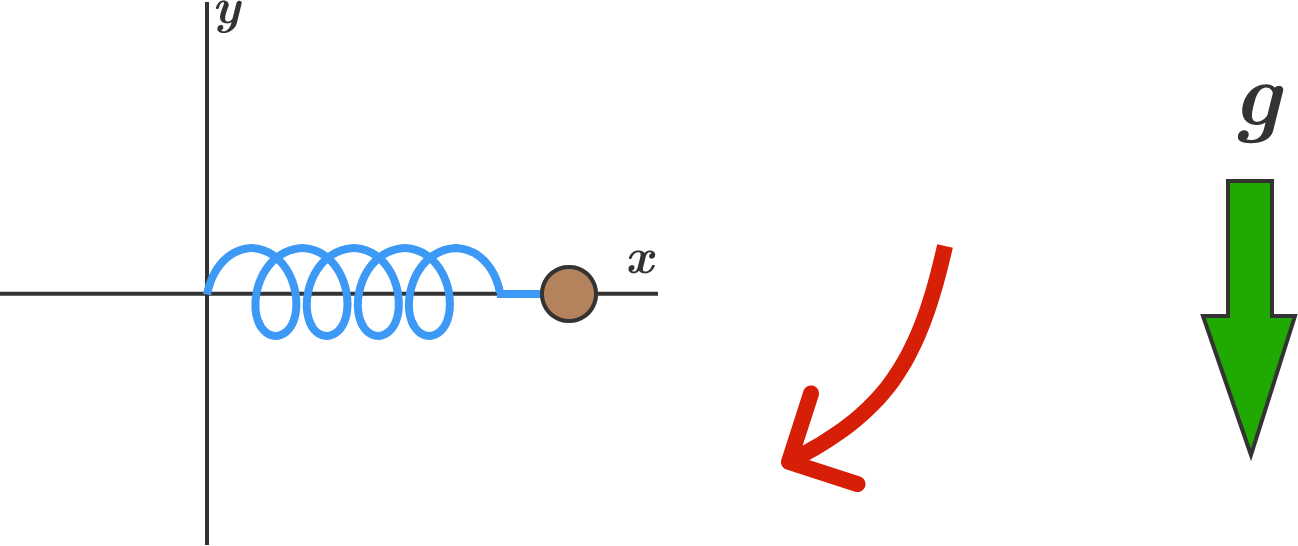# Rotating Mass-Spring SystemIn the $xy$-coordinate system, a $\SI{1}{\kilo\gram}$ mass is attached to one end of a massless ideal spring. The other end of the spring is fixed at the origin. The spring constant is $k = \SI[per-mode=symbol]{10}{\newton\per\meter}$, and the spring's unstretched length is $\SI{1}{\meter}$.

The mass is initially being held in the air horizontally at $(x,y) = (\SI{1}{\meter}, \SI{0}{\meter}),$ and then is released so that gravity pulls it downwards. How far is the mass from the origin right at the moment it first crosses the (vertical) $y$-axis?


Details and Assumptions:

• There is an ambient downward gravitational acceleration of $\SI[per-mode=symbol]{10}{\meter\per\second\squared}$.